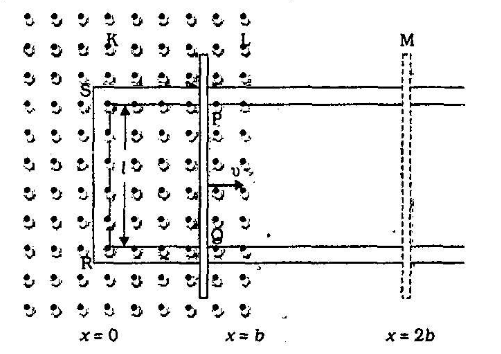Home/Class 12/Physics/The arm $$\mathit{PQ}$$  of the rectangular conductor is moved from $$x=0$$ , outwards. The uniform magnetic field is perpendicular to the plane and extends from $$x=0$$  to $$x=b$$  and is zero for $$x>b$$ . Only the arm $$\mathit{PQ}$$  possesses substantial resistance   $$r$$ . Consider the situation when the arm $$\mathit{PQ}$$  is pulled outwards from $$x=0$$  to $$x=2b$$ , and is then moved back to $$x=0$$  with constant speed $$v$$ . Obtain expressions for the flux, the induced $$\mathit{emf}$$ , the force necessary to pull the arm and the power dissipated as joule heat. Sketch the variation of these quantities with distance.Speed
00:00
10:05## QuestionPhysicsClass 12The arm $$\mathit{PQ}$$  of the rectangular conductor is moved from $$x=0$$ , outwards. The uniform magnetic field is perpendicular to the plane and extends from $$x=0$$  to $$x=b$$  and is zero for $$x>b$$ . Only the arm $$\mathit{PQ}$$  possesses substantial resistance   $$r$$ . Consider the situation when the arm $$\mathit{PQ}$$  is pulled outwards from $$x=0$$  to $$x=2b$$ , and is then moved back to $$x=0$$  with constant speed $$v$$ . Obtain expressions for the flux, the induced $$\mathit{emf}$$ , the force necessary to pull the arm and the power dissipated as joule heat. Sketch the variation of these quantities with distance.

Let the arm $$\mathit{PQ}$$  is at distance $$^\prime x^\prime$$  at any instant of time from $$x=0$$ . Then
The flux linked with the circuit $$\mathit{SPQR}$$
$${\therefore}\varphi _B=\mathit{Blx}$$ ` $$0{\leq}x\lt b$$
$$=\mathit{Blb}$$   $$b{\leq}x<2b$$
The induced $$\mathit{emf}$$  due to motion is given by:
$${\therefore}\varepsilon =\frac{-d\varphi _B}{\mathit{dt}}$$
$$=\mathit{Blv}$$ $$0{\leq}x\lt b$$
$$=0$$ $$b{\leq}x<2b$$
The induced current
$${\therefore}I=\frac{\varepsilon } r=\frac{\mathit{Blv}} r$$
In presence of magnetic field, the magnetic force on the arm $$\mathit{PQ}$$ ,
$${\therefore}F=\mathit{BIl}$$
$$=\frac{B^2l^2v} r$$   $$0{\leq}x\lt b$$
$$=0$$   $$b{\leq}x<2b$$
The power dissipation
$$\therefore \;P=I^2r$$
$$=\frac{B^2l^2v^2} r$$   $$0{\leq}x\lt b$$
$$=0$$   $$b{\leq}x<2b$$